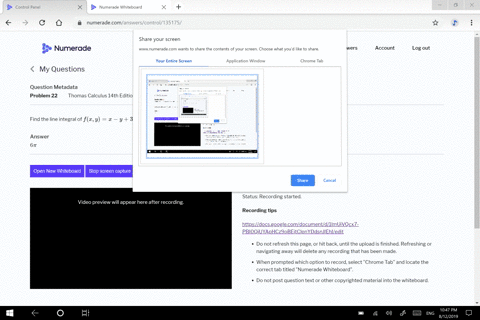🎉 The Study-to-Win Winning Ticket number has been announced! Go to your Tickets dashboard to see if you won! 🎉View Winning Ticket## Discussion

You must be signed in to discuss.

## Video Transcript

Okay, let's go ahead and step through the process of how to find, um, a line in a girl for the function f of eggs. Why equal to why Times e raise toothy X squared along a curve represented by r of T equal to four t I minus three t j and T is going to go from negative one Teoh to inclusive. Okay, So, um, let's don't forget the integral role. Um, of the function x y d s s o that line integral is represented by the integral over the curve of the function and D s with respect to S and D s is given by the magnitude of this V of tea time C t. And we want to go ahead and replace the function in terms of tea as well. So we do know that V of tea is given by the derivative of our and so we take that derivative and that will give me four I minus three j. And then the magnitude of e of T is equal to the square root of four squared. Plus that negative three squared, which is gonna give me a five and so D s is equal to five gt. Okay? And so this is going to give me the integral, uh, from negative. Wanted to of, um why is, um, negative three t? So this is going to give me a native three tea? Um, e raised to, um four t square to this is gonna be Give me a 16 t squared times five d t. Okay, so let some clean this up, and so this is going to give me a, um, negative. 15 times the integral from negative 1 to 2 of tea e raised to the 16 t square TT. Okay, so let's go ahead and kind of work with this, you know, we're gonna have to, um, so this was negative. 15 and a girl from negative 1 to 2 of t e raised to the 16 t Square DT. So we'll let you be, um, 16 t square. So d'you is equal to 32 tea. T t. All I have is a t t t. So we're in. Divide that 32 over. And so, um, 1/32 to use equal to two t d t. So I'm a substitute somewhere. Bring in. Um, 1/32 take him to the outside on the integral. And so let's go ahead and change our limits as well. So when tea is negative one. Yeah. You is going to be, um, 16. And when t is equal to two, Um, this is, um you will be 64. And so I have e to the u do you okay? And so, um e the integral of heat of the U. S. Just eat of the U. And we're evaluating it at 64 16. So this becomes negative. 15 over 32 um, e to the 64 minus e to the 16. Um, which we can actually change if we want that 15/32 to be positive, it's gonna be eating the 16 minus eat of the 64. And so there is, um, evaluating that